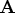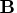Previous Page | Next Page

 Language Reference

Concatenation Operator, Horizontal:   ||

concatenates matrices horizontally

matrix1||matrix2

The horizontal concatenation operator (||) produces a new matrix by horizontally joining matrix1 and matrix2. Matrix1 and matrix2 must have the same number of rows, which is also the number of rows in the new matrix. The number of columns in the new matrix is the number of columns in matrix1 plus the number of columns in matrix2.

For example, the following statements produce the matrix, as shown:

```
a={1 1 1,
7 7 7};
b={0 0 0,
8 8 8};
c=a||b;
```

```
C             2 rows      6 cols    (numeric)

1         1         1         0         0         0
7         7         7         8         8         8
```
Now, suppose
```
b={A B C,
D E F};
c={"GH" "IJ",
"KL" "MN"};
```
In this case, the following statement produces the matrix, as shown:
```
a=b||c;
```

```
A        2 rows      5 cols    (character, size 2)

A  B  C  GH IJ
D  E  F  KL MN
```

For character operands, the element size in the result matrix is the larger of the two operands. In the preceding example,has element size 2.

You can use the horizontal concatenation operator when one of the arguments has no value. For example, ifhas not been defined andis a matrix,||results in a new matrix equal to.

Quotation marks (") are needed around matrix elements only if you want to embed blanks or maintain uppercase and lowercase distinctions.Previous Page | Next Page | Top of Page